# Summary of Analyzing Motion of Systems of Particles Civil Engineering (CE) Notes | EduRev

## Civil Engineering (CE) : Summary of Analyzing Motion of Systems of Particles Civil Engineering (CE) Notes | EduRev

The document Summary of Analyzing Motion of Systems of Particles Civil Engineering (CE) Notes | EduRev is a part of the Civil Engineering (CE) Course Introduction to Dynamics and Vibrations- Notes, Videos, MCQs.
All you need of Civil Engineering (CE) at this link: Civil Engineering (CE)

3.4 Review of concepts for Chapter 3

3.4.1 Concept checklist

Here are the skills that you should develop based on the material in this chapter:

• Be able to idealize an engineering design as a set of particles, and know when this idealization will give accurate results
• Choose an appropriate set of geometric variables to describe the motion of a system of particles (eg components in a fixed coordinate system; components in a polar coordinate system, etc)
• Be able to differentiate position vectors (with proper use of the chain rule!) to determine velocity and acceleration; and be able to integrate acceleration or velocity to determine position vector.
• Be familiar with simple harmonic motion and definitions of amplitude, frequency and period
• Be able to describe motion in normal-tangential and polar coordinates (eg be able to write down vector components of velocity and acceleration in terms of speed, radius of curvature of path, or coordinates in the cylindrical-polar system).
• Be able to convert between Cartesian to normal-tangential or polar coordinate descriptions of motion
• Be able to draw a correct free body diagram showing forces acting on system idealized as particles
• Understand and be able to describe mathematically the forces exerted by springs and dampers, and draw forces exerted by springs/dampers on a free body diagram.
• Be able to write down Newton’s laws of motion in rectangular, normal-tangential, and polar coordinate systems
• Be able to obtain an additional moment balance equation for a rigid body moving without rotation or rotating about a fixed axis at constant rate.
• Be able to use Newton’s laws of motion to solve for unknown accelerations or forces in a system of particles
• Use Newton’s laws of motion to derive differential equations governing the motion of a system of particles
• Solve the differential equations of motion analytically using Mupad, for cases where analytical solutions are available
• Be able to re-write second order differential equations as a pair of first-order differential equations in a form that MATLAB can solve
• Write a MATLAB script to solve differential equations governing the motion of a system

Of course, these are all just tricks of the trade. They are supposed to help you design a system that does something useful; or to understand (and ultimately to predict) the behavior of some physical or biological system.

3.4.2 Summary of main equations and definitions

Position-velocity-acceleration relations in a Cartesian Frame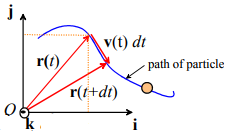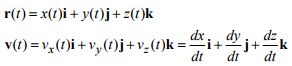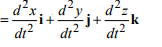The direction of the velocity vector is tangent to its path.

The magnitude of the velocity vector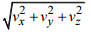is the distance traveled along the path per unit time (speed).

A unit vector tangent to the path can be found as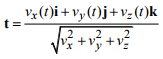Straight line motion with constant acceleration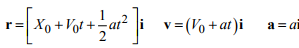Here, a is the (constant) acceleration; X0,V0, are the position and speed at time t=0.

Straight line motion with time/position dependent acceleration

Acceleration given as a function of time: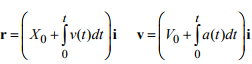Acceleration given as a function of position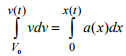Separation of variables for one-dimensional motion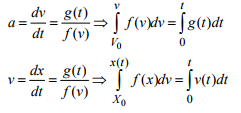Simple Harmonic Motion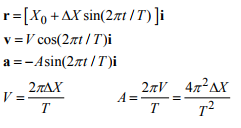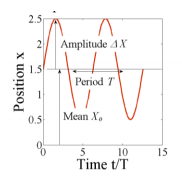Circular Motion at Constant Speed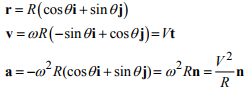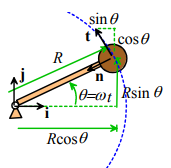General Circular Motion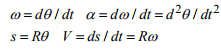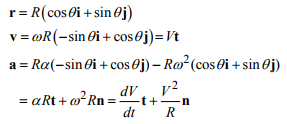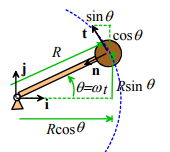Note that the straight-line motion relations can be used to relate θ,ω,α by exchanging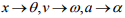Motion along an arbitrary path in normal-tangential coordinates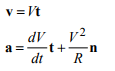For a path with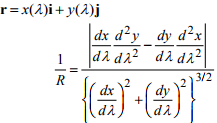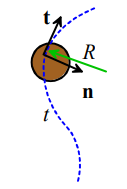Position-velocity-acceleration relations in polar-coordinates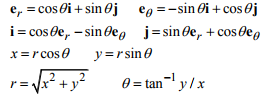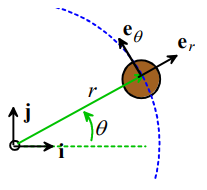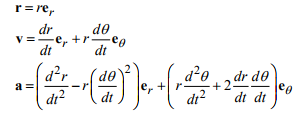Newton’s laws

For a particle F = ma

For a rigid body moving without rotation or rotating at fixed angular rate about a fixed axis MC = 0 (you must take moments about the center of mass)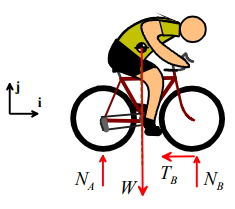Drawing free body diagrams:

1. Decide which part of a system you will idealize as a particle (you may need more than one particle)
2. Draw the part of the system you have idealized as a particle by itself (very important!). It is important to make sure that your particle is isolated – it can’t be touching something else. You may need more than one drawing if you have more than one particle in your system.
3. Draw on any of the following external forces that apply. Make sure you draw them acting in the correct direction, acting on the correct part of the body:
a. gravity (at the COM);
b. air resistance or lift forces (and sometimes moments) – various conventions are used to locate these forces but in this course we usually put them at the COM and neglect moments;
c. Buoyancy forces (act at the COM of the displaced fluid)
d. Electrostatic or electromagnetic forces

4. Draw the forces exerted by springs attached to the particle. It is best to assume that springs always pull on the point they are connected to, and that the magnitude of the force in the spring is Fs  = k(l -l0) (where l is the length of the spring, and l0 is its unstretched length.
5. Draw the forces exerted by dashpots or dampers (like springs, assume they pull on the object they are connected to, and exert a force magnitude Fd = λdl / dt  where l is the length of the dashpot.
6. Draw forces exerted by cables. Cables always pull, and exert a force parallel to the direction of the cable. The magnitude of the force has to be left as an unknown.
7. Draw any unknown reaction forces, with the following rules:
a. Reaction forces must act at any point on any point of the body that is touching something outside the particle (i.e. a part of your system that you did not include in your drawing in step 2).
b. If the connection between the two touching objects prevents them from rotating with respect to one another (or, like a motor, makes them rotate with some controllable angular speed), you will need to draw both reaction forces and moments. (Reaction moments do sometimes come up in dynamics problems, but they are not very common, so think carefully before including them).
c. If friction acts at the contact point, and you don’t know whether the two objects slide at the contact (or you know they do not slide), draw both a normal and a tangential force with unknown magnitudes N,T (or some suitable variable). The direction of the friction force is not important. DO NOT assume T = μN.
d. If friction acts at the contact point, and you know the contact slips, draw both a tangential and a normal force. You must draw the tangential force so that it opposes the direction of sliding (ask a faculty member or TA if you don’t understand this). If slip occurs you can assume T = μN.
e. If the contact point is frictionless, draw only a normal force.
f. If your particle is being touched by a two-force member (no, this is not a gender and sexuality class… a two force member is a massless rod, connected through freely rotating hinges at both ends. A massless freely rotating wheel can also be idealized as a two-force member) you can assume the reaction force acts parallel to the twoforce member.
g. If you have more than one particle in your system, make sure that any forces exerted by one particle on the other have equal and opposite reactions

Calculating unknown forces or accelerations using Newton’s laws:

1. Decide how to idealize the system (what are the particles?)
2. Draw a free body diagram showing the forces acting on each particle
3. Consider the kinematics of the problem. The goal is to calculate the acceleration of each particle in the system – you may be able to start by writing down the position vector and differentiating it, or you may be able to relate the accelerations of two particles (eg if two particles move together, their accelerations must be equal).
4. Write down F=ma for each particle.
5. If you are solving a problem involving a massless frames (see, e.g. Example 3, involving a bicycle with negligible mass) you also need to write down MC = 0 about the particle.
6. Solve the resulting equations for any unknown components of force or acceleration (this is just like a statics problem, except the right hand side is not zero).

Problems like this will usually ask you to make some design prediction at the end, which might involve calculating critical conditions for something to slip, tip, break, etc.

• At the onset of slip at a contact |T| = μ|N|
• At the critical point where an object tips over, a reaction force somewhere will go to zero. You will have to identify where this point is, find the reaction force, and set it to zero.

Deriving equations of motion for a system of particles

1. Introduce a set of variables that can describe the motion of the system. Don’t worry if this sounds vague – it will be clear what this means when we solve specific examples. 2. Write down the position vector of each particle in the system in terms of these variables
3. Differentiate the position vector(s), to calculate the velocity and acceleration of each particle in terms of your variables;
4. Draw a free body diagram showing the forces acting on each particle. You may need to introduce variables to describe reaction forces. Write down the resultant force vector. 5. Write down Newton’s law F = ma for each particle. This will generate up to 3 equations of motion (one for each vector component) for each particle.
6. If you wish, you can eliminate any unknown reaction forces from Newton’s laws. If you are trying to solve the equations by hand, you should always do this; of you are using MATLAB, it’s not usually necessary – you can have MATLAB calculate the reactions for you. The result will be a set of differential equations for the variables defined in step (1) 7. If you find you have fewer equations than unknown variables, you should look for any constraints that restrict the motion of the particles. The constraints must be expressed in terms of the unknown accelerations.
8. Identify the initial conditions for the variables defined in (1). These are usually the values of the unknown variables, their time derivatives, at time t=0. If you happen to know the values of the variables at some other instant in time, you can use that too. If you don’t know their values at all, you should just introduce new (unknown) variables to denote the initial conditions.
9. Solve the differential equations, subject to the initial conditions.

Trajectory equations for particle moving near earth’s surface with no air resistance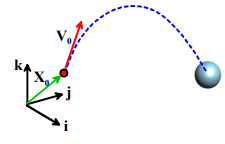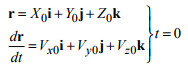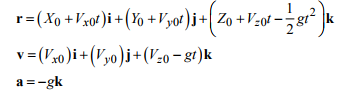Example: to solve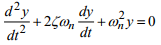with initial conditions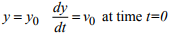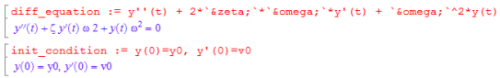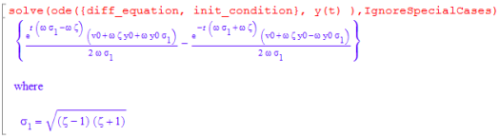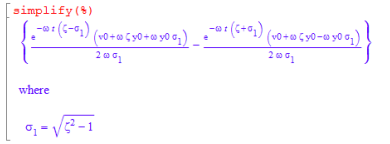Re-writing a second-order differential equation as a pair of first-order equations for MATLAB

Example: to solve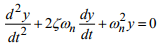we introduce v = dy / dt as an additional variable. This new equation, together with the original ODE can then be written in the following form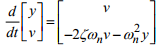This is now in the form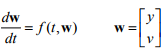as required.

Offer running on EduRev: Apply code STAYHOME200 to get INR 200 off on our premium plan EduRev Infinity!

## Introduction to Dynamics and Vibrations- Notes, Videos, MCQs

20 videos|53 docs

,

,

,

,

,

,

,

,

,

,

,

,

,

,

,

,

,

,

,

,

,

;